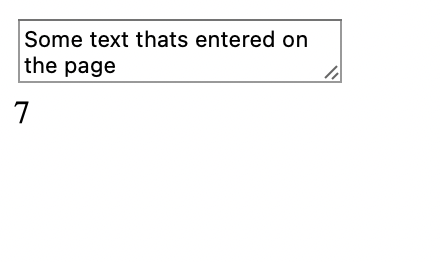# JavaScript: Count Words in a String

If you have a String object in JavaScript and you want to know the number of words it has, you can achieve this in various ways, lets see some of the code examples.

#### Example 1: Reading textarea data and finding the number of words in it.

``````<textarea id="myData" onBlur="getWordCount();"></textarea>
<div id="count"></div>
<script>

function getWordCount() {
var myString = document.getElementById("myData").innerHTML;
document.getElementById("count").innerHTML = myString.split(" ").length;
}
/<script>``````
Output:JavaScript String Word Count Example
JSFiddle: https://jsfiddle.net/k12yzp5v/

As you can see in the above example we have called a function onBlur of a textarea and read the text as a variable and then called two operations over it. First, we have done split based on space to get the array of words and then used length to get the count.

In the first example there are many ways we can go wrong. It's always better to use the trim() function along with the first example as there might be cases where the first and the last text in the string are spaces and we may get the wrong count, also what if there are multiple spaces between two words.

Example 2: Using regular expressions (RegEx)

Using regular expression is another way of knowing the number of words in a string, lets see an example,

``````var string = 'This is some random String to get its connt';
console.log(string.match(/\w+/g).length);``````
Output:

9

JSFiddle: https://jsfiddle.net/u80fx3pa/

Have Questions? Post them here!